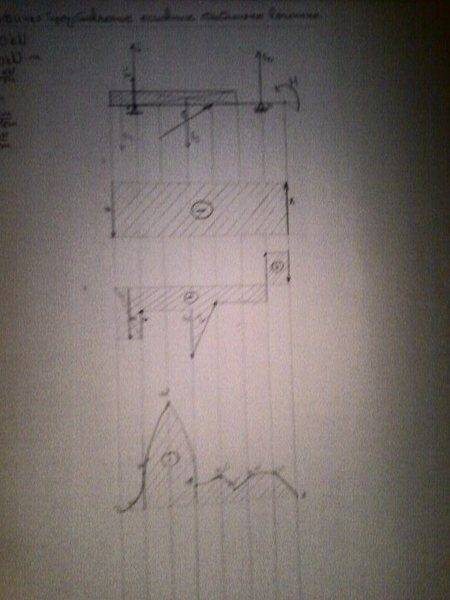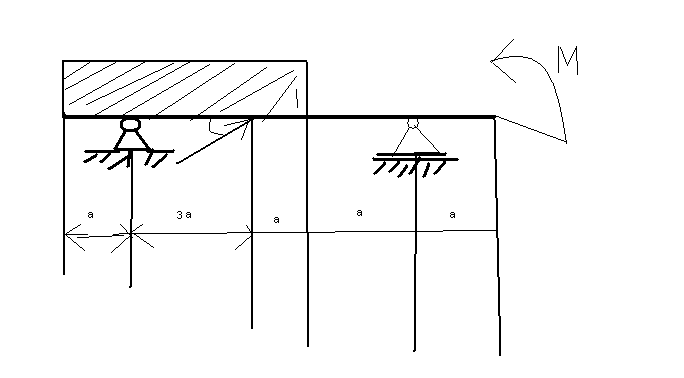# Mechanics problem.

## Homework Statement

For carrier loaded the way it's shown in the picture and whose load-values are:
F=50kN, M=20kNm, g= 10kN/m and a=1m
a) find analytically resistances of supporters
b) find analytically intensities of elementary static dimensions for section(cut) at the point C on the carrier
c)find the greatest moment of flexion
d)graphically represent the check of axial forces, transversal forces and moments of flexion

## The Attempt at a Solution

a) resistance of supporters:
##\sum _{i=1} ^n X_i = 0 \\ X_A + X = 0 \\ X_A = -X \\ X_A = -43.25kN \\ \\ \sum _{i=1} ^n Y_i = 0 \\ Y_A - F_g + F_B - Y = 0 \\ \\ \sum _{i=1} ^n M_A = \\ -Y6a + F_B5a - M - F_ga = 0 \\ F_B = \frac{Y6a + M + F_ga}{5a} \\ F_B=\frac{25*6*1 + 20 + 40*1}{5*1} \\ F_B = 42kN \\ \\ Y_A = F_g - F_B + Y \\ Y_A = 40 - 42 + 25 \\ Y_A = 23kN \\ \\ F_A = \sqrt{x_A^2 + Y_A^2} \\ F_A = 48.98kN \\ \\ tan\alpha_A = \frac{Y_A}{X_A} \\ tan\alpha_A = -o.531 \\ \alpha_{A'} = 62\degree 60'##

b)
## F_A= - X_A = 43.25kN \\ F_t = Y_A - g3a = -7kN \\ M_X = Y_A2a - g3a = 16kNm##

c) ##D: M_X = 0 \\ E: M_X = g\frac{a}{2}3a^4 = 15kNm \\ A: M_X = 9*3a^4 = 30kNm \\ F: M_X = 92a^2 + Y_A3a = 89kNm \\ C: M_X = Y_A2a - g3a = 16kNm \\ G: Y_Aa^3 = 23kNm \\ H':\frac{a}{2}a^2 + M = 10kNm \\ H'': M_X = a^2 + M = 20kNm \\ B: M_X = a + M = 21kNm \\ J: M_X = 0 ##

and now graphically (picture of carrier is also shown here) :Now, i would like to know if i made any mistakes here.

SteamKing
Staff Emeritus
Homework Helper
This image is so blurry as to be useless for checking your work. Please post a better image or make a separate sketch of the problem.Is it ok?

SteamKing
Staff Emeritus
Homework Helper
30 degrees

SteamKing
Staff Emeritus
Homework Helper

## Homework Statement

For carrier loaded the way it's shown in the picture and whose load-values are:
F=50kN, M=20kNm, g= 10kN/m and a=1m
a) find analytically resistances of supporters
b) find analytically intensities of elementary static dimensions for section(cut) at the point C on the carrier
c)find the greatest moment of flexion
d)graphically represent the check of axial forces, transversal forces and moments of flexion

## The Attempt at a Solution

a) resistance of supporters:
##\sum _{i=1} ^n X_i = 0 \\ X_A + X = 0 \\ X_A = -X \\ X_A = -43.25kN \\ \\ \sum _{i=1} ^n Y_i = 0 \\ Y_A - F_g + F_B - Y = 0 \\ \\ \sum _{i=1} ^n M_A = \\ -Y6a + F_B5a - M - F_ga = 0 \\ F_B = \frac{Y6a + M + F_ga}{5a} \\ F_B=\frac{25*6*1 + 20 + 40*1}{5*1} \\ F_B = 42kN \\ \\ Y_A = F_g - F_B + Y \\ Y_A = 40 - 42 + 25 \\ Y_A = 23kN \\ \\ F_A = \sqrt{x_A^2 + Y_A^2} \\ F_A = 48.98kN \\ \\ tan\alpha_A = \frac{Y_A}{X_A} \\ tan\alpha_A = -o.531 \\ \alpha_{A'} = 62\degree 60'##
I've checked your calculations of the reactions at A and B.

It's not clear from the diagram, but I'm assuming that the beam is pinned at A and free to move horizontally at B, which means that A can resist a force applied axially.
In that case, the horizontal reaction at A would be -43.25 kN as you indicated.

For the vertical reactions, it seems you have written in incorrect moment equation about point A. Therefore, the vertical reactions you have calculated are incorrect.

Remember, the distributed load w and the vertical component of the concentrated load F are working in opposite directions from one another, that is, the vertical component of the concentrated load F is tending to support the beam and reduce the reactions at A and B.

You ask us to tell you if there are mistakes. As far as the reactions are concerned this is something that you could check yourself. In this case, having obtained the reactions, by summing forces in x and y directions, plus taking moments about A (wherever that is - not annoted), you do the check by taking moments about any point other than A, and if there is equilibrium, then either you are correct or you have self-cancelling errors. The same is true for functions such as bending moment. If you use the definition of bending moment that it is the algebraic sum of moments on one side of a section, then this should be precisely the same as the algebraic sum of the moments on the other side of the section; and that is your check. errors are usually caused by mis-applying sign conventions. The same applies to shear force or axial force. So you don't need US to check your work when you could do it yourself, and this is what people in industry have to do when they are analyzing real world problems not found in text books.